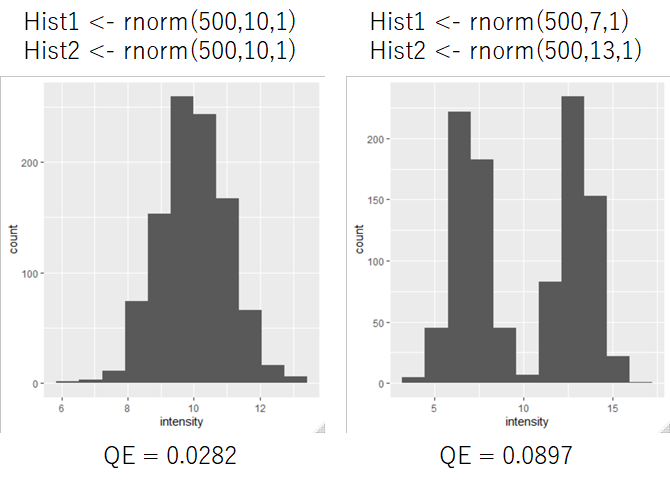# Calculate Rao's Quadratic Entropy (QE) to evaluate cell-cell heterogeneity for HCA

High Content Analysis (HCA) is one of the powerful tools for the drug discovery.

High-content screening (HCS), also known as high-content analysis (HCA) or cellomics, is a method that is used in biological research and drug discovery to identify substances such as small molecules, peptides, or RNAi that alter the phenotype of a cell in a desired manner.
High-content screening - Wikipedia

HCA can detect the single cell phenotype and measure many phenotype information (target intensity, size&shape, and texture) , however, in most cases, single-cell data is averaged per well to simplify analysis.
Advanced Assay Development Guidelines for Image-Based High Content Screening and Analysis - Assay Guidance Manual - NCBI Bookshelf

Some researchers tried to detect cell-cell heterogeneity in high content analysis. Identifying and Quantifying Heterogeneity in High Content Analysis: Application of Heterogeneity Indices to Drug Discovery
Biologically Relevant Heterogeneity: Metrics and Practical Insights

Rao's Quadratic Entropy (QE) were used as index of cellular diversity in this paper.

Rao's quadratic entropy is a measure of diversity of ecological communities defined by Rao (1982)
https://rdrr.io/cran/SYNCSA/man/rao.diversity.html
https://www.sciencedirect.com/science/article/pii/0040580982900041They evaluated potential indices of Diversity and showed that QE (Quadratic entropy) increase steadily with two different sample histogram distribution.
https://doi.org/10.1371/journal.pone.0102678.s007

I 'm very interesting to calculate Quadratic Entropy, so I calculate QE of model distributions by using R.

```library(ggplot2)

min <- 0
max <- 20

hist1 <- rnorm(500,10,1)
hist2 <- rnorm(500,10,1)
hist <- c(hist1, hist2)
data <- data.frame(intensity = hist)

#Calculate number of bins
len <- length(data\$intensity)
K <- 1 + log2(len)

plt <- ggplot(data,aes(x=intensity))+
geom_histogram(bins=round(K))

plt

#Cut the data to each bin based on the braks
data\$bins <- cut(data\$intensity, breaks=break_data,label=FALSE)

data <- na.omit(data)
hist_data <- data.frame(table(data\$bins))

result <- data.frame(Num = seq(1:length(break_data)))
#Merge result and hist_data by left outer join
result <- merge(result,hist_data,by.x="Num", by.y ="Var1",all=T)
result[is.na(result)] <- 0

#Calculate Frequency
result\$"Freq" <- result\$"Freq"/sum(result\$"Freq")
#Normalized Number
result\$"Num" <- (result\$"Num"-min(result\$"Num"))/(max(result\$"Num")-min(result\$"Num"))

#Calculate distance
distance <-  dist(result\$"Num",method = "euclidean")

D <- as.matrix(distance)
p <- as.vector(result\$"Freq")